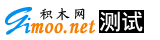# 单元测试的小技巧介绍和举例

' returns the sum of the two numbers
Function Sum(ByVal a As Integer, ByVal b As Integer) As Integer

<TestMethod()> _
Dim result As Integer = Sum(1, 2)
End Sub

TDD中一个好的测试要求你去修改代码，从而使它能够按照想要的方式工作，这一点要胜于强迫你去反映现在的真实情况或者一个非逻辑要求的渴望的结果。例如，当1＋1返回0时就意味着测试失败。这个简单的例子和这种情况是相似的，在练习中，如果现在的需求是在工作的，测试应该可以反映你所期待的结果，然后你可以调整现在代码的情况去通过这个测试。

1.?在旧的需求或者无效或者在别处测试的情况下删除被验证本质上不再有效的失败的测试
2.?改变旧的测试使你可以测试新的要求（本质上使用新的测试），然后在新的设置下（测试的逻辑状态相同，但是初始功能函数可能有所不同）测试旧的需求。

Public Function Sum(ByVal x As Integer, ByVal y As Integer, _
?ByVal allowNegatives As Boolean) As Integer
If Not allowNegatives Then Throw New Exception()

Return x + y
End Function

' Try this...
If Not True Then ' replace flag with const
?If x < 0 OrElse y < 0 Then Throw New Exception()
End If

' Or this...
If Not allowNegatives Then

?' replace check with const
If False OrElse y < 0 Then Throw New Exception()
End If

<TestMethod()> _
Public Sub Sum_AnyParamBiggerThan1000IsNotSummed()
Assert.AreEqual(3, Sum(1001, 1, 2)
Assert.AreEqual(3, Sum(1, 1001, 2) ' Assert fails
Assert.AreEqual(3, Sum(1, 2, 1001) ' This line never executes
End Sub

<TestMethod()> _
Public Sub TestCalcParseNegative()
Dim c As New Calc
?Assert.AreEqual(1000, c.Parse("-1, -1000")
End Sub

<TestMethod()> _
Public Sub Parse_NegativeFirstNum_ReturnsSumOfTheRestAsPositive()
Dim c As New Calc
Dim parsedSumResult As Integer = c.Parse("-1", "-1000")
?Const SUM_WITH_IGNORED_FIRST_NUM As Integer = 1000
Assert.AreEqual(SUM_WITH_IGNORED_FIRST_NUM, parsedSumResult)
End Sub

Figure 5 展现了一个拥有两个成员变量的类的测试。但是他们中的一个(cxNum)只被部分使用。Figure 6 展现了如何在测试中替换代码从而使它更加易读的方法。